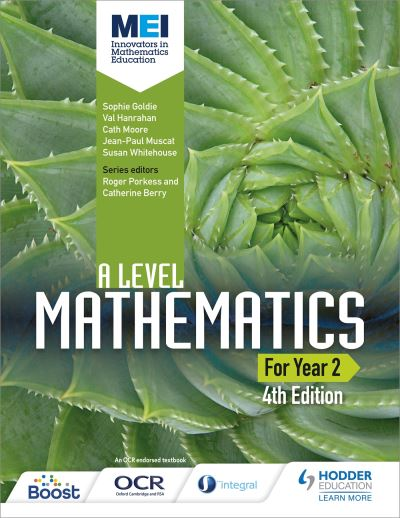# MEI A Level Mathematics for Year 2: student book : fourth edition

Series: MEI

Format: Paperback

ISBN: 9781471852985

Publication Date: 28 Jul 2017

Subject: Mathematics

Suitable for: Secondary (Year 12, Year 13)

Encourage every student to develop a deeper understanding of mathematical concepts and their applications with textbooks that draw on the well-known MEI (Mathematics in Education and Industry) series, updated and tailored to the 2017 OCR (MEI) specification and developed by subject specialists and MEI.

### £24.89

RRP £29.99 Save 17%

Quantity

• Our review
• Details
Notes
to view our full review.

Summary

Encourage every student to develop a deeper understanding of mathematical concepts and their applications with textbooks that draw on the well-known MEI (Mathematics in Education and Industry) series, updated and tailored to the 2017 OCR (MEI) specification and developed by subject specialists and MEI.

Endorsed for OCR.

-Develop problem-solving, proof and modelling skills with plenty of
questions and well-structured exercises that build skills and
mathematical techniques.
-Build connections between topics, using real-world contexts to help
develop mathematical modelling skills, thus providing a fuller and more
coherent understanding of mathematical concepts.
-Prepare students for assessment with practice questions written by
subject specialists.
-Ensure coverage of the new statistics requirements with five dedicated
statistics chapters and questions around the use of large data sets.
-Supports the use of technology with a variety of questions based around
the use of spreadsheets, graphing software and graphing calculators.
-Provide clear paths of progression that combine pure and applied maths
into a coherent whole.
-Reinforce Year 1 content with short review chapters.

Contents

Getting the most from this book
Prior knowledge

1 Proof
1 Problem solving
2 Methods of proof
2 Trigonometry
2 Circular measure
3 Small-angle approximations
Review: Algebra 1
1 Surds and indices
2 Exponentials and logarithms
3 Sequences and series
1 Definitions and notation
2 Arithmetic sequences and series
3 Geometric sequences and series
Review: Algebra 2
1 Equations and inequalities
2 Polynomials
4 Functions
Review: Graphs and transformations
1 The language of functions
2 Composite functions
3 The modulus function
5 Differentiation
Review: Differentiation
1 The shape of curves
2 The chain rule
3 Connected rates of change
4 The product and quotient rules
Practice questions: Pure Mathematics 1
Review: The sine and cosine rules
1 Working with triangles
Problem solving: Triples
6 Trigonometric functions
1 Reciprocal trigonometric functions
2 Working with trigonometric equations and identities
7 Further algebra
Review: Pascal's triangle and the binomial expansion
1 The general binomial expansion
2 Simplifying algebraic expressions
3 Partial fractions
8 Trigonometric identities
1 Compound angle formulae
2 Double angle formulae
3 The forms rcos (q ± a), rsin(q ± a)
9 Further differentiation
1 Differentiating exponentials and logarithms
2 Differentiating trigonometric functions
3 Implicit differentiation
10 Integration
Review: Integration
1 Finding areas
2 Integration by substitution
3 Integrating other functions
4 Integration involving the natural logarithmic function
5 Further integration by substitution
6 Integration by parts
Practice questions: Pure Mathematics 2
Review: Coordinate geometry
1 Line segments
2 Circles
Problem solving: Eggs
11 Parametric equations
1 Graphs from parametric equations
2 Finding the equation by eliminating the parameter
3 Parametric differentiation
12 Vectors
1 Vectors
2 Using vectors to solve problems
13 Differential equations
1 First order differential equations
2 Solving differential equations by separating the variables
14 Numerical methods
1 Solving equations numerically
2 The Newton-Raphson method
3 Numerical integration
Problem solving: Numerical integration
Practice questions: Pure Mathematics 3
Review: Working with data
1 Statistical problem solving
Problem solving: Trains
15 Probability
Review
1 The probability of events from two experiments
2 Conditional probability
16 Statistical distributions
Review
1 Discrete random variables
2 The Normal distribution
17 Statistical hypothesis testing
Review
1 Interpreting sample data using the Normal distribution
2 Bivariate data: correlation and association
Practice questions: Statistics
18 Kinematics
Review
1 Motion in two or three dimensions
19 Forces and motion
Review
1 Forces in equilibrium
2 Finding resultant forces
3 Newton's second law in two dimensions
20 Moments of forces
1 Rigid bodies
21 Projectiles
1 Equations for projectile motion
2 Projectile problems
3 Further examples
4 The path of a projectile
5 General equations
Problem solving: Fireworks and aeroplanes
22 A model for friction
1 A model for friction
Practice questions: Mechanics

Index
Acknowledgements

Category
NF
Subject
Mathematics
Dewey
510
Interest level
to view interest age.
Series
Publisher
Pub. date
28 Jul 2017
Edition
Original
ISBN
9781471852985
BIC code
Binding
Paperback
Pages
592
Width
213mm
Height
276mm
Weight
1624g
Index
Yes
Contents page
Yes
to view levelling information.

This site uses cookies. By continuing to browse the site you are agreeing to our use of cookies. Review our cookies information for more details.